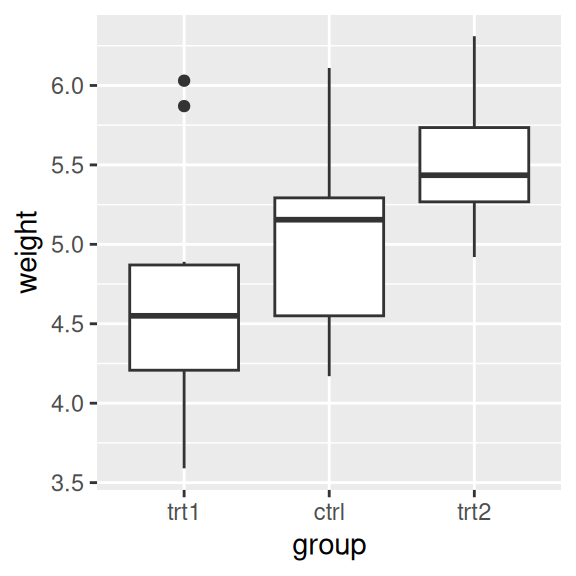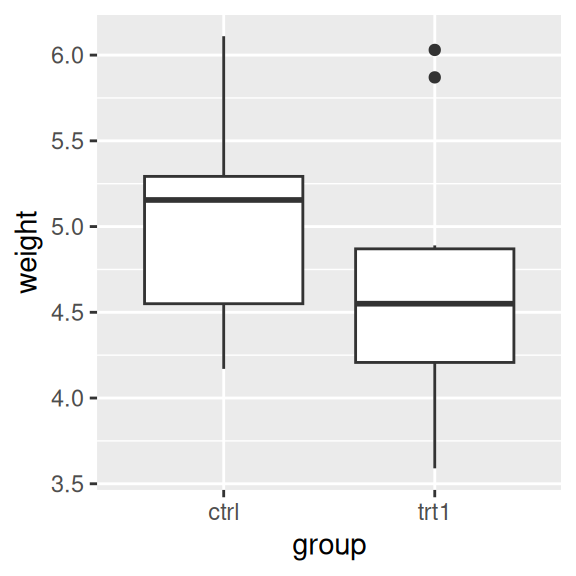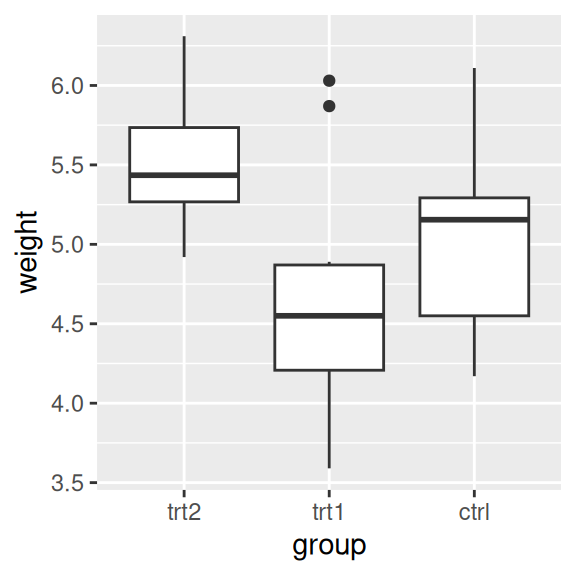## 8.4 Changing the Order of Items on a Categorical Axis

### 8.4.1 Problem

You want to change the order of items on a categorical axis.

### 8.4.2 Solution

For a categorical (or discrete) axis – one with a factor mapped to it – the order of items can be changed by setting limits in `scale_x_discrete()` or `scale_y_discrete()`.

To manually set the order of items on the axis, specify limits with a vector of the levels in the desired order. You can also omit items with this vector, as shown in Figure 8.8, left:

``````pg_plot <- ggplot(PlantGrowth, aes(x = group, y = weight)) +
geom_boxplot()

pg_plot +
scale_x_discrete(limits = c("trt1", "ctrl", "trt2"))``````

### 8.4.3 Discussion

You can also use this method to display a subset of the items on the axis. This will show only `ctrl` and `trt1` (Figure 8.8, right). Note that because data is removed, it will emit a warning when you do this.

``````pg_plot +
scale_x_discrete(limits = c("ctrl", "trt1"))
#> Warning: Removed 10 rows containing missing values (stat_boxplot).``````Figure 8.8: Box plot with manually specified items on the x-axis (left); With only two items (right)

To reverse the order, set `limits = rev(levels(...))`, and put the factor inside. This will reverse the order of the `PlantGrowth\$group` factor, as shown in Figure 8.9:

``````pg_plot +
scale_x_discrete(limits = rev(levels(PlantGrowth\$group)))``````Figure 8.9: Box plot with order reversed on the x-axis